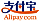Buchcover von Pythagorean Trigonometric Identity
Buchtitel:

Pythagorean Trigonometric Identity

Betascript Publishing (30.09.2013 )gutscheinfähig
ISBN-13:

978-613-0-30642-7

ISBN-10:
6130306423
EAN:
9786130306427
Buchsprache:
Englisch
Klappentext:
Please note that the content of this book primarily consists of articles available from Wikipedia or other free sources online. The Pythagorean trigonometric identity is a trigonometric identity expressing the Pythagorean theorem in terms of trigonometric functions. Along with the sum-of-angles formulae (see Angle sum and difference identities) it is the basic relation among the sin and cos functions from which all others may be derived. If the trigonometric functions are defined in terms of the unit circle, the proof is immediate: given an angle θ, there is a unique point P on the unit circle centered at the origin in the Euclidean plane at an angle θ from the x-axis, and cos θ, sin θ are respectively the x- and y-coordinates of P. By definition of the unit circle, the sum of the squares of these coordinates is 1, hence the identity.
Verlag:
Betascript Publishing
Webseite:
https://www.betascript-publishing.com/
Herausgegeben von:
Lambert M. Surhone, Mariam T. Tennoe, Susan F. Henssonow
Seitenanzahl:
124
Veröffentlicht am:
30.09.2013
Lagerbestand:
Lieferbar
Kategorie:
Mathematik
Preis:
39,00 €
Stichworte:
List of Trigonometric Identities, Pythagorean Theorem, Trigonometric Functions, Right Triangle, Power Series, Unit circle, Differential Equation, TrigonometryKategorien